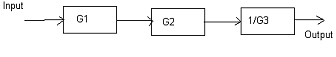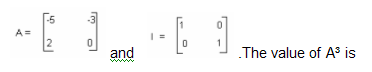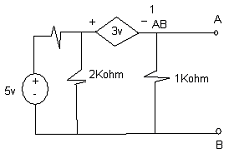# Electrical Engineering test questions for exams and entrance - 6

## Electrical Engineering test questions for exams and entrance - 6

(1) Let T be the time period of a periodic signal x (t) and for some time “t?” let y (t) = x (t - to) + x (t + to). Let b denotes the Fourier series coefficients of y (t). For all odd

values of k if b? = 0 then “to” can be equal to

(A) T/2
(B) 2T
(C) T
(D) T/4

(2) The figure shows three subsystems connected in cascade. G1, G2 and 1/G3 are the respective gains of the system and ε1, ε2 and ε3 are the respective relative small

errors associated with each respective subsystem. The error associated with the output is(A) ε1+ ε2 + ε3
(B) 1/ε1+ ε2 + ε3
(C) ε1+ ε2 - ε3
(D) ε1- ε2 + ε3

(3) Consider a system 2/(s+1). For a step response to reach 98% of its final value, the approximate time taken will be

(A) 1s
(B) 4s
(C) 10s
(D) 8s

(4) The logic gates that are designated as universal gates are

(A) AND, NAND and OR gates
(B) NOR and NAND gates
(C) AND, OR and NOT gates
(D) XNOR, NOR and NAND gates

(5) Single slope integrating type and successive approximation type are the two 8-bit ADC’s and to convert 5v analog input signal into equivalent digital output they take time Ta and Tb

respectively. If the input analog signal is reduced to 2.5v then the approximate time taken by the two ADC’s will be respectively

(A) Ta/2, Tb
(B) Ta, Tb/2
(C) Ta, Tb
(D) Ta/2, Tb/2

(6) For the signal h (t) the Fourier transform is H (jω) = (2cosω)(sin2ω)/ ω. The value of h (0) is

(A) 1/2
(B) 1/4
(C) 2
(D) 1

(7) For the signal x (t) = 8 sin (0.8πt+π/4) the period is

(A) 0.8s
(B) 2.5s
(C) 1s
(D) 0.8πs

(8) In a certain alternator a field excitation of 20 A results in an armature current of 400 A in short circuit and a terminal voltage of 2000V on open circuit. At a load current of 200A,

the magnitude of the internal voltage drop within the machine is

(A) 100V
(B) 10V
(C) 1000V
(D) 300V

(9) The leakage flux in an induction motor is

(A) The flux that links the stator winding or the rotor winding but not both
(B) The flux that links both the stator winding and the rotor winding
(C) The flux that does not link any winding
(D) The flux that leaks through the machine.

ANSWER: The flux that links the stator winding or the rotor winding but not both

For Questions 10 and 11 refer to the data given below:

While supplying the rated load at a speed of 80 rad/sed, a 240 V dc shunt motor draws 15 A current. Armature resistance = 0.5 Ω and field winding resistance = 80 Ω.

(10) At the time of plugging, the net voltage across the armature resistance will be

(A) 230V
(B) 324V
(C) 300V
(D) 474V

(11) To limit the armature current to 125% of its rated value, the external resistance to be added in the armature circuit is

(A) 6.4 Ω
(B) 10.6 Ω
(C) 16.2 Ω
(D) 31.1 Ω

(12) A 3-phase synchronous motor is connected to an ac main. The motor runs at full load and unity power factor. With field current held constant if its shaft load is reduced by half, its

new power factor will be

(A) Unity
(B) Lagging
(D) Dependent on machine parameters.

(13) Consider a unity feedback linear system. The steady state error of this system for a unit step input is 0.1. What will be the steady state error of the same system for a pulse input

whose magnitude is 10 and duration is of 1sec?

(A) 0
(B) 1
(C) 0.1
(D) 0.5

(14) What is the common use of negative sequence relay?

(A) To protect a transformer
(B) To protect an alternator
(C) To protect a bus bar
(D) To protect a transmission line

(15) When a triangular wave symmetric about the time-axis is fed to an average reading digital multimeter, it reads 10 V. The rms reading meter for the same input will read

(A) 10/√3
(B) 10√2
(C) 20/√3
(D) 20√3

(16) There are two switches for a bulb, one switch at ground floor and the other one at the first floor. The bulb can be turned ON or OFF by any one of the switched irrespective of the

state of the other switch. This logic of switching of bulb resembles

(A) An OR gate
(B) A NAND gate
(C) An AND gate
(D) An XOR gate

(17) What will be the content of accumulator after the instructions given below is executed in an 8085 microprocessor?

XRA A
MVI, B F0H
SUB B

(A) 10 H
(B) 01 H
(C) 0F H
(D) F0 H

(18) A maximum frequency of 5Khz of a band-limited signal is to be sampled. The sampling frequency in KHz, which is not valid according to sampling theorem, is

(A) 20
(B) 15
(C) 5
(D) 10

(19) A non-magnetic core has a mean circumference of 300mm and cross-sectional area of 300mm². A coil of 300 turns is wound on it. What will be the inductance of the coil

corresponding to a magnetizing current of 3A?

(A) 132.01 μH
(B) 154.23 μH
(C) 101.32 μH
(D) 113.04 μH

(20) The function f (t) = sin t / t at t=0 has

(A) A minima
(B) A maxima
(C) A discontinuity
(D) A point of inflection

(21) The solution of the differential equation t dx/dt + x = t with initial condition x(1) = 0.5 is

(A) x = t
(B) x = t²/2
(C) x = t/2
(D) x = 2t

(22)(A) 15A + 13I
(B) 15A + 15I
(C) 19A + 30I
(D) 19A + 19I

(23) A salient pole alternator whose armature resistance is negligible has direct axis reactance of 1.2p.u and quadrature axis reactance of 1.0p.u. What is the power angle of this

alternator if it is delivering rated kVA at upf and at rated voltage?

(A) 45°
(B) 90°
(C) 120°
(D) 60°

For Questions 24 and 25 refer to the diagram given below:(24) The Thevenin’s equivalent resistance across the terminal A and B is

(A) 0.5Kohm
(B) 0.11Kohm
(C) 0.2Kohm
(D) 1Kohm

(25) The Thevenin’s equivalent voltage across the terminal A and B is

(A) 1.5V
(B) 0.25V
(C) 0.5v
(D) 1V